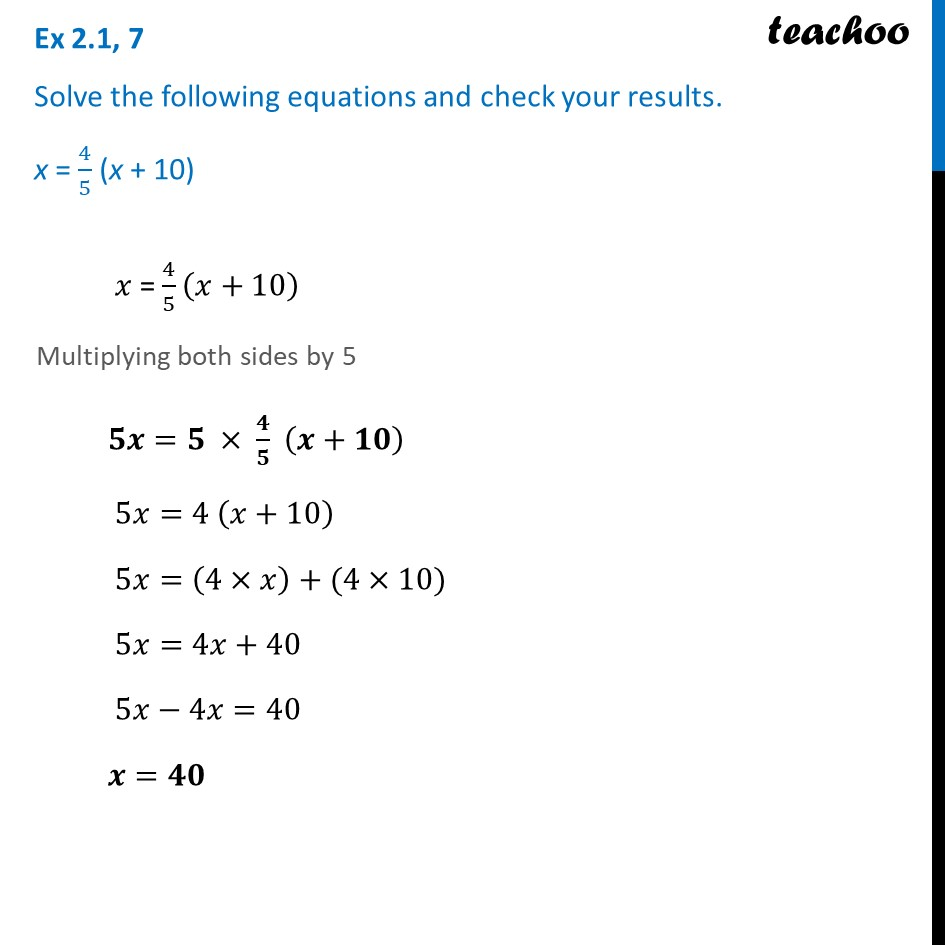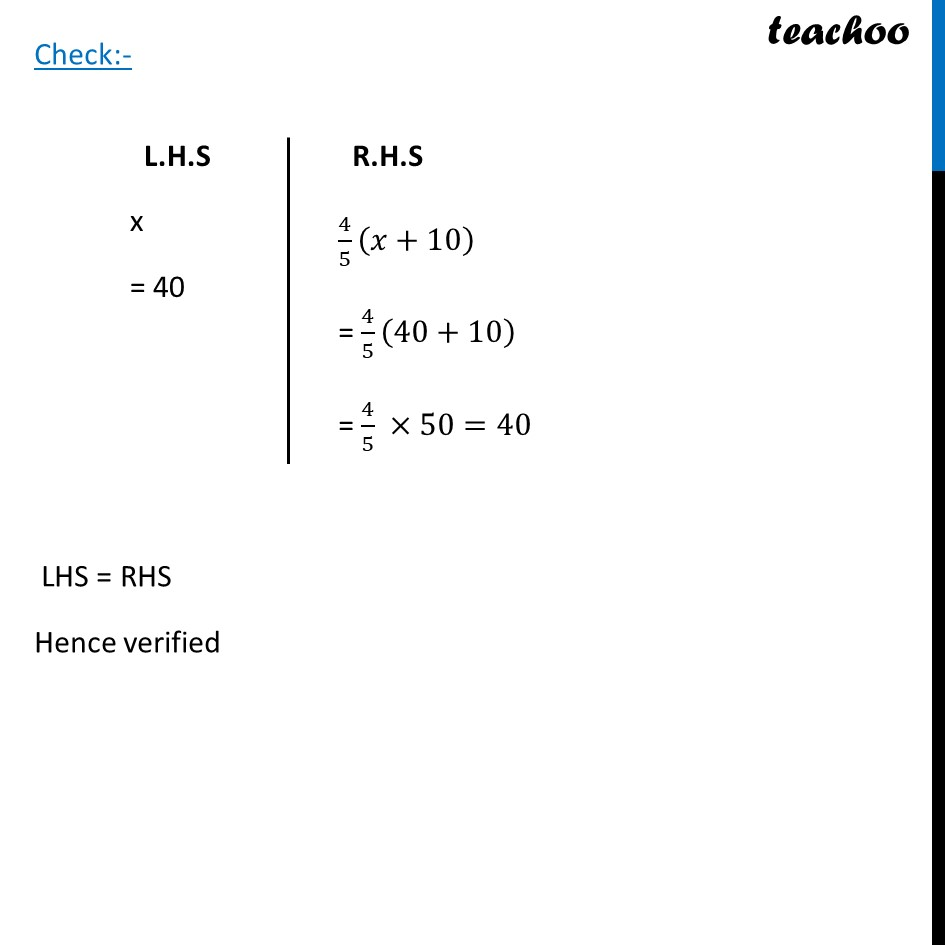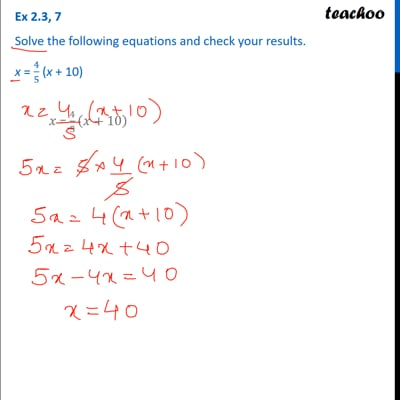Ex 2.1

Chapter 2 Class 8 Linear Equations in One Variable
Serial order wiseThis video is only available for Teachoo black users

Learn in your speed, with individual attention - Teachoo Maths 1-on-1 Class

### Transcript

Ex 2.1, 7 Solve the following equations and check your results. x = 4/5 (x + 10) 𝑥 = 4/5 (𝑥+10) Multiplying both sides by 5 𝟓𝒙=𝟓 × 𝟒/𝟓 (𝒙+𝟏𝟎) 5𝑥=4 (𝑥+10) 5𝑥=(4×𝑥)+(4×10) 5𝑥=4𝑥+40 5𝑥−4𝑥=40 𝒙=𝟒𝟎 L.H.S x = 40 R.H.S 4/5 (𝑥+10) = 4/5 (40+10) = 4/5 ×50=40 Check:- LHS = RHS Hence verified